# How to Design an LED Backlight Driving System using the MAX20446

### 要約

This application note details a step-by-step design process for the MAX20446 6-Channel Backlight High Brightness LED driver and highlights the calculations needed to speed up the selection of critical components. The trade-offs of component selection are also discussed. This application note focuses on the boost converter topology, and the same design process can be extended to other LED driver products.

#### Introduction

The MAX20446 is a peak current-mode-controlled LED driver, capable of driving up to six LED strings in several different configurations: boost, buck-boost, SEPIC, and flyback topologies. This application note focuses on the boost topology in which the LED string forward voltage is always higher than the input supply voltage range.

The MAX20446 offers the following features:

• Six integrated current outputs that can sink up to 120mA LED current each
• Integrated spread-spectrum and phase shifting
• I2C-controlled pulse-width modulation (PWM) dimming and hybrid dimming
• Programmable switching frequency between 400kHz and 2.2MHz.

For this LED backlight driving system design example, six 7-LED strings are driven with a constant current of 100mA each. Assume that each LED has a maximum expected forward-voltage drop of 3.3V and minimum expected forward-voltage drop of 2.7V. Also assume that the LED driver circuit is directly fed by the car battery, which has a typical VIN of 12V but can vary from 5V to 16V.Figure 1. Typical MAX20446 operating circuit.

#### Inductor Selection

To select the right inductor value, calculate the required total output current (ILED) to drive the LED using the following equation: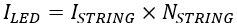(Eq. 1)

where ISTRING is the current per string, and NSTRING is the number of strings used.

The maximum voltage (VLED_MAX) to drive the LED strings is given by the following equation: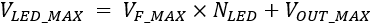(Eq. 2)

where VOUT_MAX is the maximum OUT_ regulation voltage (1.1V from the MAX20446 data sheet’s Electrical Characteristics), VF_MAX is the maximum expected forward drop on each LED, and NLED is the number of LEDs that form each string.

The maximum duty cycle (DMAX) is given by the following equation:(Eq. 3)

where VLED_MAX is the forward voltage of the LED string in volts, VD is the forward drop of the rectifying diode (approximately 0.6V), VIN_MIN is the minimum input-supply voltage in volts, VCS is the peak current sense voltage in volts (90% of the available limit should be considered), and VFET is the average drain-to-source voltage of the switching MOSFET in volts when it is on (assume 0.1V initially).

The maximum duty cycle and LED current determine the average inductor current (ILAVG), which is expressed by the following equation: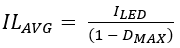(Eq. 4)

Knowing the average inductor current, the peak inductor current (ILP) is expressed as follows:(Eq. 5)

where ?IL is the peak-to-peak inductor current ripple in amperes. A lower ripple current requires a larger (and typically more expensive) inductor. A higher ripple current not only leads to higher switching losses but also requires more slope compensation and increased input capacitance. If the maximum peak-to-peak ripple is the recommended ±30% of the average inductor current, ?IL is given by the following equation:(Eq. 6)

From Equation 4, the average inductor current is proportional to the output current, and because the inductor ripple current, ?IL, is independent of output load current, the minimum and the maximum values of the inductor current track the average inductor current exactly. Based on this, Equation 5 can be rewritten as follows: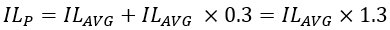(Eq. 7)

Finally, the minimum inductance value (LMIN) in Henrys is expressed by the following equation:(Eq. 8)

where fSW is the desired switching frequency in hertz and LTOL is the tolerance parameter applied on the inductance’s nominal value.

As an example, if fSW = 2.2MHz, LTOL = 30% and VCS = 0.378V, the values are calculated as follows:(Eq. 9)(Eq. 10)(Eq. 11)(Eq. 12)(Eq. 13)(Eq. 14)(Eq. 15)

When the minimum inductor value is determined, a real inductor value must be chosen that is as close as possible to LMIN without going under. Recalculate the peak inductor current and ripple using the chosen inductor value. These numbers are necessary for additional calculations going forward.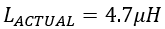(Eq. 16)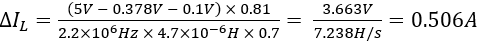(Eq. 17)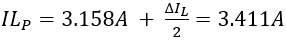(Eq. 18)

Ensure that the chosen inductor has a current rating higher than ILP. Typically, 20% headroom is used for the inductor peak current.

#### Input Capacitor Selection

In a boost converter, the input current is continuous, so the input capacitor’s RMS ripple current is low. Both bulk capacitance and ESR contribute to the input ripple. Assume equal ripple contributions from bulk capacitance and ESR if aluminum electrolytic and ceramic capacitors are both used in parallel. If only ceramic capacitors are used, most of the input ripple comes from the bulk capacitance (since ceramic capacitors have very low ESR). Use Equation 19 and Equation 20 to calculate the minimum input bulk capacitance and maximum ESR.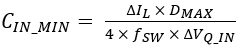(Eq. 19)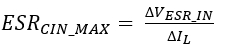(Eq. 20)

where ?VQ_IN and ?VESR_IN are the input voltage ripple contributions due to capacitor discharge and ESR respectively.

Assume that a maximum input ripple of 50mV can be tolerated (1% of VIN_MIN) where 95% of this input ripple comes from the bulk capacitance, and calculate the input capacitor as follows:(Eq. 21)(Eq. 22)

Considering a 20% tolerance on the capacitor’s nominal value, use a 4.7µF capacitor to achieve the 0.98µF minimum bulk capacitance. Ensure that the chosen capacitors meet the minimum bulk capacitance requirement at the operating DC voltage (capacitance can decrease substantially with a change in voltage in ceramic capacitors).

The dielectric material should be X7R or better. Otherwise, the capacitor can lose much of its capacitance due to DC bias or temperature. The total capacitance value can also be increased if the input voltage is noisy or to meet electromagnetic interference (EMI) requirements.

#### Output Capacitor Selection

In the boost converter topology, the output capacitor supplies the load current when the switching MOSFET is on. The function of the output capacitor is to reduce the converter output ripple to acceptable levels. The entire output-voltage ripple appears across the constant-current sink outputs because the LED-string voltages are stable due to the constant current.

The ESR, ESL, and the bulk capacitance of the output capacitors contribute to the total output voltage ripple. In most applications, using low-ESR ceramic capacitors can dramatically reduce the output ESR and ESL effects. To reduce the ESL and ESR effects, connect multiple ceramic capacitors in parallel to achieve the required bulk capacitance. To attenuate audible noise during PWM dimming, the number of ceramic capacitors on the output is usually minimized.

In this case, an additional electrolytic or aluminum organic polymer capacitor provides most of the bulk capacitance. Alternatively, low acoustic noise ceramic capacitors can be used.

Determine the minimum bulk capacitance at the boost output by using the following equation:(Eq. 23)

where ?VQ_OUT is the output voltage ripple contribution due to capacitor discharge.

An additional output voltage ripple contribution (?VESR_OUT) comes from the output capacitor ESR, which is given by the following equation: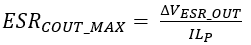(Eq. 24)

Limit the peak-to-peak output voltage ripple to 50mV to get a stable output current. Assume that the bulk capacitance is responsible for 95% of the ripple contribution, and calculate the following values using Equation 23 and Equation 24.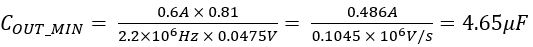(Eq. 25)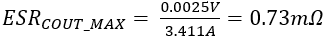(Eq. 26)

Use three 4.7µF ceramic capacitors in parallel to exceed the minimum output capacitance of 4.65µF because the chosen capacitors must meet the minimum bulk capacitance requirement at the operating voltage.

#### Overvoltage Protection

If any LED string is open, the DC-DC converter output voltage increases to achieve the desired LED current. The overvoltage-protection threshold limits the output voltage through a voltage-divider network connected between the converter output, the BSTMON input, and GND. If the BSTMON voltage exceeds 1.23V, NDRV is forced low, which turns off the switching MOSFET and prevents the boost output voltage from increasing.

The overvoltage-protection threshold at the DC-DC converter output is determined using the following equation:(Eq. 27)

where 1.23V (typ) is the overvoltage threshold on BSTMON.

VBSTMON is the maximum voltage the boost converter can produce and should be greater than the maximum expected LED string voltage (VLED_MAX) according to the following inequality:(Eq. 28)

where the factor 1.1 takes into account a 10% margin.

The minimum expected LED string voltage (VLED_MIN) is expressed by the following equation:(Eq. 29)

where VOUT_MIN = 0.7V.

If undervoltage occurs during startup, the boost converter latches off. To avoid the boost converter from latching off, the voltage on the BSTMON pin must always be greater than 0.6V. This results in the following relation between VBSTMON and VLED_MIN:(Eq. 30)

The inequalities from Equation 28 and Equation 30 can be combined to yield the following:(Eq. 31)

Select values for RBSTMON1 and RBSTMON2 so that the output voltage does not exceed its absolute maximum rating (52V) while respecting Eq. 31. By selecting RRBSTMON1 = 226k? and RBSTMON2 = 10k?, the following value for VBSTMON is obtained:(Eq. 32)

#### Slope Compensation and Current-Sense Resistors Selection

The MAX20446 is a current-mode-controlled LED driver, which means that information about the inductor current is fed back into the loop.

At duty cycles greater than 50% and with continuous (i.e., always greater than zero) inductor current, a load transient can cause subharmonic oscillation and loop instability without slope compensation. To keep the loop stable, a resistor (RSC) must be added from CS to the source of the switching MOSFET. Internal to the MAX20446, there is a current source that feeds a small ramped current through RSC to create a voltage on the slope-compensation resistor (VSC). This voltage is added to the voltage across the FET current-sense resistor, RCS_FET, and the result is compared to a reference voltage, namely the voltage on the COMP pin.

Because RCS_FET has both the switching MOSFET current and the slope compensation current flowing through it, the total voltage on the CS pin is expressed by the following equation: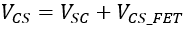(Eq. 33)

The slope compensation voltage is defined as follows:(Eq. 34)

To maintain stability, the minimum amount of slope-compensation voltage rate needed is expressed by the following equation:(Eq. 35)

where IL_UPSLOPE and IL_DOWNSLOPE are expressed as follows: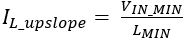(Eq. 34)(Eq. 36)

Therefore, VSC_MIN and RSC_MIN are defined by the following equations:(Eq. 37)(Eq. 38)

This includes a 1.5 factor to provide adequate margin.

Recalling Equation 33, the minimum value of the RCS_FET resistor is obtained by solving the following equation:(Eq. 39)

where 0.39V is the minimum value of the peak current-sense threshold voltage. The current-sense threshold also includes the slope-compensation component. The minimum current-sense threshold of 0.39V is multiplied by 0.9 to take tolerances into account.

RCS_FET is then expressed by the following equation: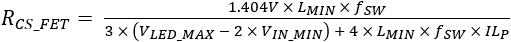(Eq. 40)

Based on the stated design specifications, the values of RCS_FET and RSC are calculated as follows: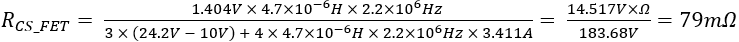(Eq. 41)(Eq. 42)

RCS_FET = 75mO is selected, which is the closest lower-value standard resistor.
RSC = 2.7k? is selected for this application.

#### Switching MOSFET Selection

The external switching MOSFET’s voltage rating should be sufficient to withstand the sum of the maximum output voltage and the rectifier diode forward drop as stated in the following equation:(Eq. 43)

The switching MOSFET should also be rated to handle the maximum RMS current:(Eq. 44)

where IDRMS is the switching MOSFET’s drain RMS current in amperes, and the factor 1.3 is included to consider a 30% margin.

For this example application, the required VDS_FET_ABS_MAX and IDRMS are calculated as follows:(Eq. 45)(Eq. 46)

The MOSFET’s on resistance (RDSON) is related to the current rating and affects the boost converter’s efficiency because it determines the resistive power loss of the device. The higher it is, the lower the overall efficiency of the converter is. Calculate the resistive power loss by using the following equation: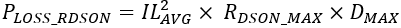(Eq. 47)

Given the total output power (POUT) and an estimated overall LED driver’s efficiency (?) of 90%, the PLOSS_TOT value can be obtained as follows: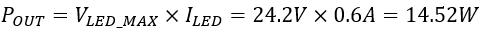(Eq. 48)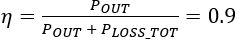(Eq. 49)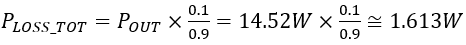(Eq. 50)

The RDSON_MAX value, which limits the PLOSS_RDSON impact on overall efficiency to 1%, can be determined by using the following equation:(Eq. 51)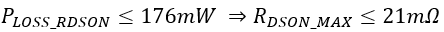(Eq. 52)

Another consideration concerns the gate charge because the gate driver must provide that charge to turn the MOSFET on and off. A smaller charge is better, and the switching speed is also important, but it does not show significant variations between MOSFETs of similar current, on resistance, and voltage ratings.

The ON Semiconductor® NVTFS5C471NL N-channel MOSFET’s characteristics are suitable for this application.

#### Rectifier Diode Selection

The rectifier diode can be a major contributor to overall power loss. Choose a Schottky diode with low forward-voltage drop that is rated to handle the average LED current. Use the following equation to determine the required current rating for the rectifier diode: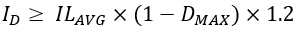(Eq. 53)

where the factor of 1.2 is included for margin.

With the previously calculated values of ILAVG = 3.158A and DMAX = 0.81, the rectifier diode is required to handle a forward current of 0.72A, as shown in Equation 54.(Eq. 54)

Also ensure that the Schottky diode has a reverse voltage rating 20% higher than VLED_MAX, the maximum expected reverse voltage across the diode.

The most evident limitations of Schottky diodes are their relatively low reverse voltage ratings and their relatively high reverse leakage current. For silicon-metal Schottky diodes, the reverse voltage is typically 50V or less. Reverse leakage current increases with temperature, which leads to a thermal instability issue that often limits the useful reverse voltage to well below the actual rating.

The ON Semiconductor NRVBS260T3G Schottky diode is selected based on the calculated results.

#### Error Amplifier Compensation

Figure 2 shows the generic open loop configuration of a current-mode boost converter operating with continuous inductor current.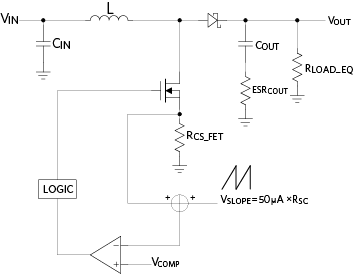Figure 2. Generic current-mode boost converter open loop configuration.

In the MAX20446 LED driver, RLOAD_EQ is replaced by.The transfer function, A(s), is given by the following equation: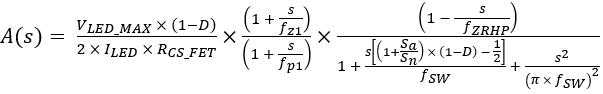(Eq. 55)

where the artificial compensation ramp slope (SA), the inductor on-slope (SN), fZ1, fRHPZ, and fP1 are expressed in the following equations:fRHPZ is the worst-case frequency at which the right-half-plane (RHP) zero is located. The RHP zero differs from the traditional left-half-plane (LHP) zero. Instead of boosting the phase, the RHP zero lags the phase further down, which reduces the phase margin and causes the loop to be unstable.

If ceramic capacitors with almost zero ESR are used at the converter’s output, fZ1 can be neglected because it shifts toward high frequencies and most likely falls out of the converter’s bandwidth.

The dominant pole’s fP1 frequency is twice a common pole frequency due to the effect of boosting. Loop compensation is guaranteed by a so-called Type II transconductance amplifier, which characterizes the closed-loop response (B(s)). Figure 3 shows the basic Type II transconductance amplifier circuit with external compensation components.Figure 3. Type II transconductance amplifier for loop compensation.

Assuming R0 >> RCOMP and CCOMP >> CHF, the B(s) transfer function of Figure 3 can be written as follows: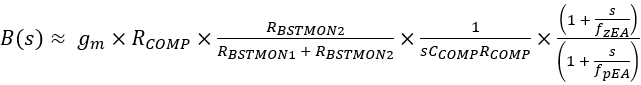(Eq. 56)

where AVM is the mid-band voltage gain and gM is the transconductance of the error amplifier. AVM is are expressed as follows:fZEA and fPEA are expressed as follows: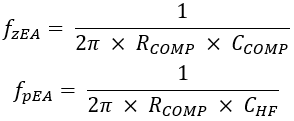The closed loop response of the LED driver is A(s) × B(s)

The goal of loop compensation is to ensure that there is less than 180 degrees of phase shift for loop gains greater than 0dB (and an adequate phase margin). The error amplifier adds a zero-frequency pole due to the integrating effect of CCOMP, which allows rolling off the loop gain to 0dB with a -20dB/decade slope (after the dominant pole and well before the effects of the RHP zero).

It is recommended that the closed loop gain crossover frequency, fC, is limited to at least one fifth of fRHPZ to obtain an acceptable phase margin that is higher than 45 degrees. The compensation zero, fZEA, should be placed at least one fifth of the target crossover frequency, which is approximately 1/25 fRHPZ.

To fix the total loop gain at fp1 so that the total loop gain crosses 0dB with -20dB/decade slope at 1/5 fRHPZ, the optimum value of RCOMP is given by the following expression:(Eq. 57)

The value of CCOMP can then be obtained from the definition of fZEA.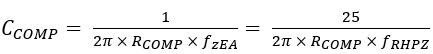(Eq. 58)

The higher the frequency that the loop gain stays above zero before crossing 0dB, the faster the loop response is and, therefore, the lower the output voltage drops during a load step. Lowering RCOMP while keeping fZEA ˜ fC/5, increases the phase margin without significantly changing the gain and increases the time it takes for the output voltage to settle following a load step.

If the total output capacitance’s ESR is significant, the output zero effect at fz1 is not negligible and can be counter-balanced by placing the error amplifier’s main pole frequency, fPEA, at fz1. Consequently, the optional CHF capacitor value is expressed as follows:(Eq. 59)

The fPEA pole might also be necessary to ensure that the gain continues to roll off after the crossover frequency.

Based on the previously calculated values for this design example, the values of fRHPZ, fP1, RLOAD_EQ, RCOMP, and CCOMP are calculated as follows:(Eq. 60)(Eq. 61)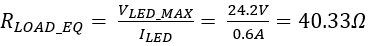(Eq. 62)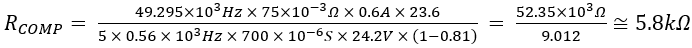(Eq. 63)(Eq. 64)

Select the standard commercial values of RCOMP = 4.7k? and CCOMP = 18nF for this numerical example to calculate FZEA as follows:(Eq. 65)

Figure 4 and Figure 5 illustrate the Bode plots of the LED driver closed loop response. A 0-dB crossing frequency (fC) of 10kHz and a phase margin (PM) of 70 degrees are obtained.Figure 4. Loop gain.Figure 5. Loop phase.

#### Conclusion

Figure 6 shows the complete boost LED driver schematic with the selected component values from the design example. The step-by-step design process outlined in this application note can be used as a reference during the debug and test phase.

For additional details, refer to the MAX20446 data sheet and the MAX20446 Evaluation Kit data sheet.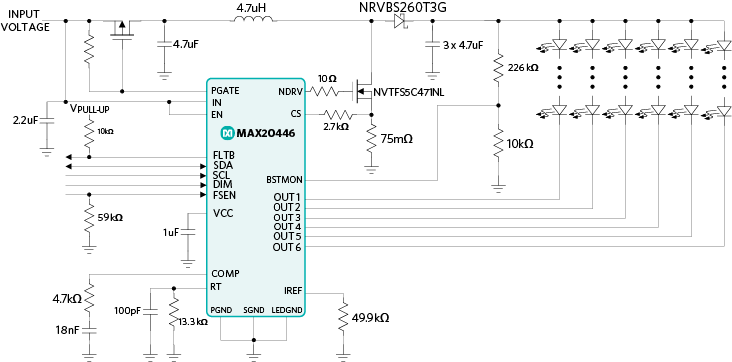Figure 6. Typical MAX20446 operating circuit based on example calculations (optional P-MOS and relevant bias resistor not included).